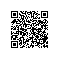# Integer.parseInt(&quot;&quot;) Integer.valueOf(&quot;&quot;)和new Integer(&quot;&quot;)之间的区别

//Integer.parseInt("")
public static int parseInt(String s) throws NumberFormatException {
return parseInt(s,10);
}
//Integer.valueOf("")
public static Integer valueOf(String s) throws NumberFormatException {
return Integer.valueOf(parseInt(s, 10));
}
//new Integer("")
public Integer(String s) throws NumberFormatException {
this.value = parseInt(s, 10);
}


public static Integer valueOf(int i) {
if (i >= IntegerCache.low && i <= IntegerCache.high)
return IntegerCache.cache[i + (-IntegerCache.low)];
return new Integer(i);
}


System.out.println(Integer.valueOf(5) == Integer.valueOf(5));
System.out.println(Integer.valueOf(500) == Integer.valueOf(500));


    false //会用到缓存
true  //不会用到缓存


• 当我们需要的是一个基本类型* int *的时候我们需要使用Integer.parseInt()函数
• 当我们需要的是一个Integer对象类的时候我们就是用Integer.valueOf()函数使用钉钉扫一扫加入圈子
+ 订阅